# A Teleseismic Event

As an example of the teleseismic record, we have selected a recent large earthquake in the Tonga region.

 Information from the USGS NEIC Location: Tonga Islands Date: 95/04/07 Origin Time (UTC): 22:06:56.89 Location: -15.199, -173.529 Depth (km): 21.2 Magnitude: 8.0 Mw

A map illustrates the location of this earthquake in the Tonga Islands, over 70 degrees away from the stations of the BDSN.

This event was well-recorded by all stations of the BDSN. This figure compares recordings from 4 sites on the same plot. These seismograms are plotted with amplitude as a function of time. These are raw waveforms and represent the recorded ground velocity on the vertical component sampled at 1 Hz (the LHZ channel). The traces are ordered by distance, with the closest station (JRSC) at the bottom and the most distant station (YBH) at the top. The traces are plotted with absolute amplitude. At these distances, the waveforms vary more slowly with distance and no single trace dominates the plot (as compared to the local example. For each station, the distance to the earthquake in degrees is labeled above the trace at the right edge of of the plot, while the maximum amplitude is labeled below.

For comparison, this figure shows the same seismograms, plotted to illustrate the variation with distance. This "record section" shows the traces at their true distance between 70 and 74 degrees. Below are three separate record sections are plotted for the three components of motion. In this case, the horizontal components have been rotated from North and East into a reference frame parallel and perpendicular to the great circle containing the earthquake and the station. In this reference frame, the "radial" component (labeled LHR) display motion in the plane between the earthquake and the station and the "transverse" component (labeled LHT) display motion perpendicular to that plane. The traces have been filtered to accentuate the low-frequency arrivals.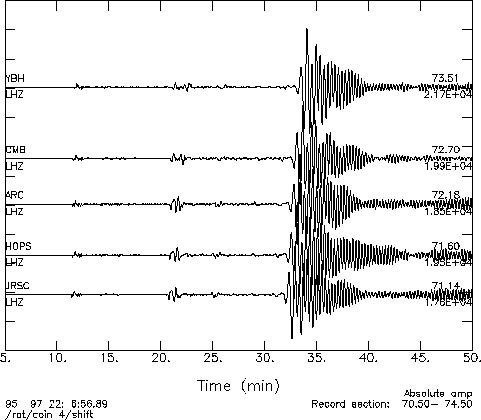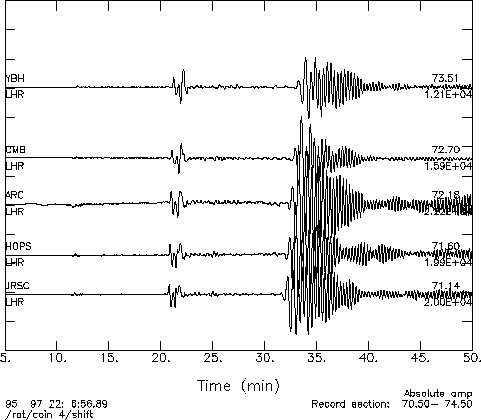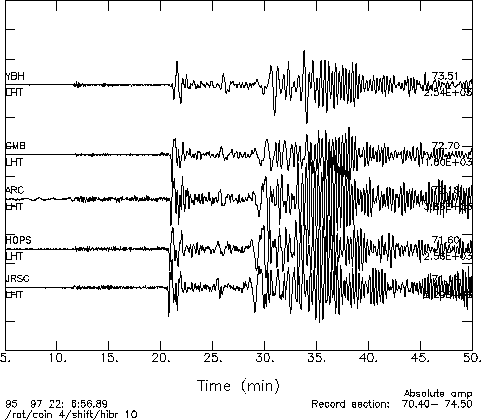The examples below include plots of the three components of ground motion (LHZ - Vertical, LHN - North, LHE - East) recorded at 5 stations. Each plot show amplitude as a function of time. Each station is shown with the same time scale and the waveforms are extremely similar from one station to another.

 Waveforms from the BDSN JRSC Distance = 71.14 degrees Azimuth = 41.05 degrees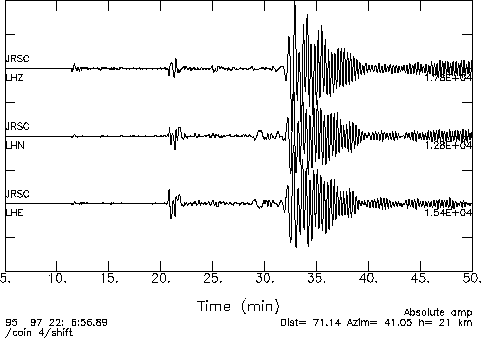HOPS Distance = 71.60 degrees Azimuth = 39.30 degrees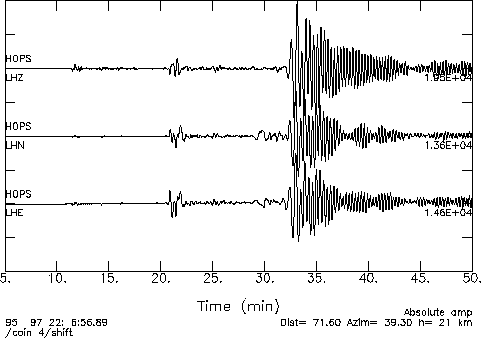ARC Distance = 72.18 degrees Azimuth = 37.25 degreesCMB Distance = 72.70 degrees Azimuth = 41.44 degreesYBH Distance = 73.51 degrees Azimuth = 37.23 degrees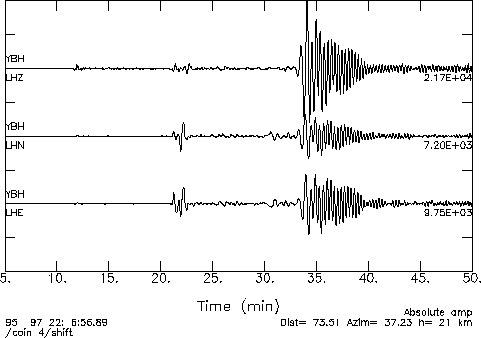View seismograms from the Global Seismic Network